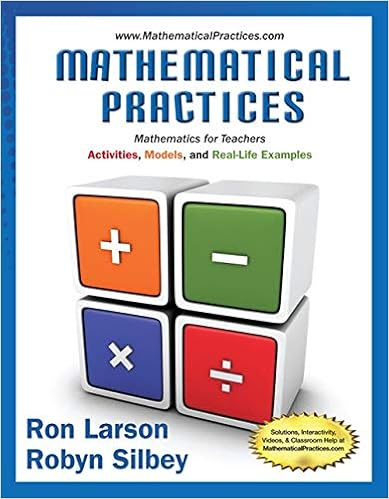# Section 3.2.pdf - 312 3.2 Chapter 3 Math Models Solve...

• 17
• 67% (3) 2 out of 3 people found this document helpful

This preview shows page 1 - 3 out of 17 pages.

##### We have textbook solutions for you!
The document you are viewing contains questions related to this textbook.The document you are viewing contains questions related to this textbook.
Chapter 7 / Exercise 119
Mathematical Practices, Mathematics for Teachers: Activities, Models, and Real-Life Examples
LarsonExpert Verified
3.2Solve Percent ApplicationsLearning ObjectivesBy the end of this section, you will be able to:Translate and solve basic percent equationsSolve percent applicationsFind percent increase and percent decreaseSolve simple interest applicationsSolve applications with discount or mark-upBe Prepared!Before you get started, take this readiness quiz.1.Convert 4.5% to a decimal.If you missed this problem, reviewExample 1.26.2.Convert 0.6 to a percent.If you missed this problem, reviewExample 1.26.3.Round 0.875 to the nearest hundredth.If you missed this problem, reviewExample 1.26.4.Multiply (4.5)(2.38).If you missed this problem, reviewExample 1.26.5.Solve3.5 = 0.7n.If you missed this problem, reviewExample 1.26.6.Subtract50 − 37.45.If you missed this problem, reviewExample 1.26.Translate and Solve Basic Percent EquationsWe will solve percent equations using the methods we used to solve equations with fractions or decimals. Without thetools of algebra, the best method available to solve percent problems was by setting them up as proportions. Now as analgebra student, you can just translate English sentences into algebraic equations and then solve the equations.We can use any letter you like as a variable, but it is a good idea to choose a letter that will remind us of what you arelooking for. We must be sure to change the given percent to a decimal when we put it in the equation.EXAMPLE 3.12Translate and solve: What number is 35% of 90?SolutionTranslate into algebra. Letn= the number.Remember "of" means multiply, "is" means equals.Multiply.31.5is35%of90TRY IT : :3.23Translate and solve:What number is 45% of 80?312Chapter 3 Math ModelsThis OpenStax book is available for free at
##### We have textbook solutions for you!
The document you are viewing contains questions related to this textbook.The document you are viewing contains questions related to this textbook.
Chapter 7 / Exercise 119
Mathematical Practices, Mathematics for Teachers: Activities, Models, and Real-Life Examples
LarsonExpert Verified
TRY IT : :3.24Translate and solve:What number is 55% of 60?
EXAMPLE 3.13Translate and solve: 6.5% of what number is \$1.17?
EXAMPLE 3.14Translate and solve: 144 is what percent of 96?
TRY IT : :3.27Translate and solve:110 is what percent of 88?TRY IT : :3.28
•••